# x(t) <---> x(s)=s^2/(s^2+5). Find the laplace transform of d^3(x(t))/dt^3 Findl the laplace tanstorm ot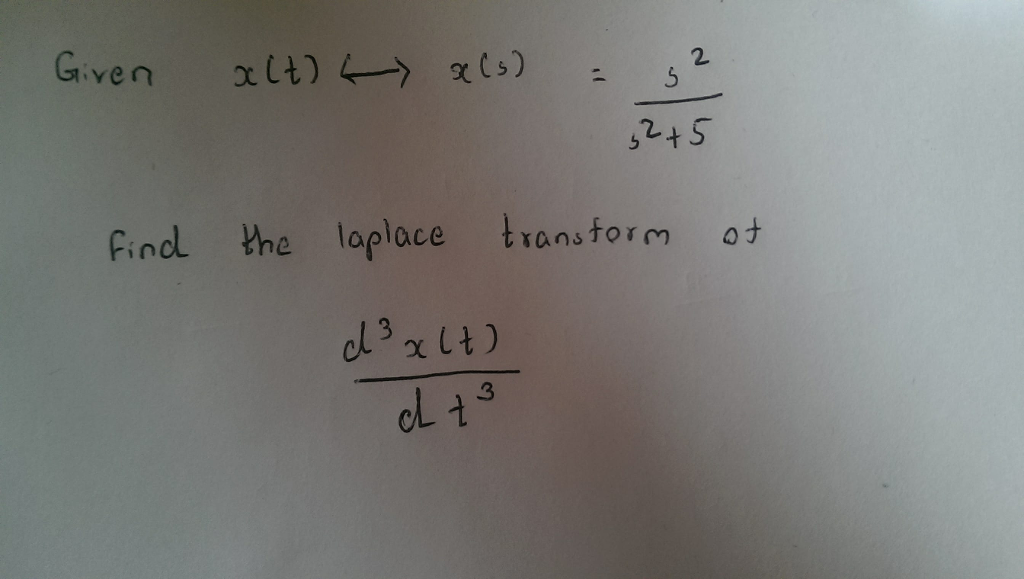x(t) <---> x(s)=s^2/(s^2+5). Find the laplace transform of d^3(x(t))/dt^3

Findl the laplace tanstorm ot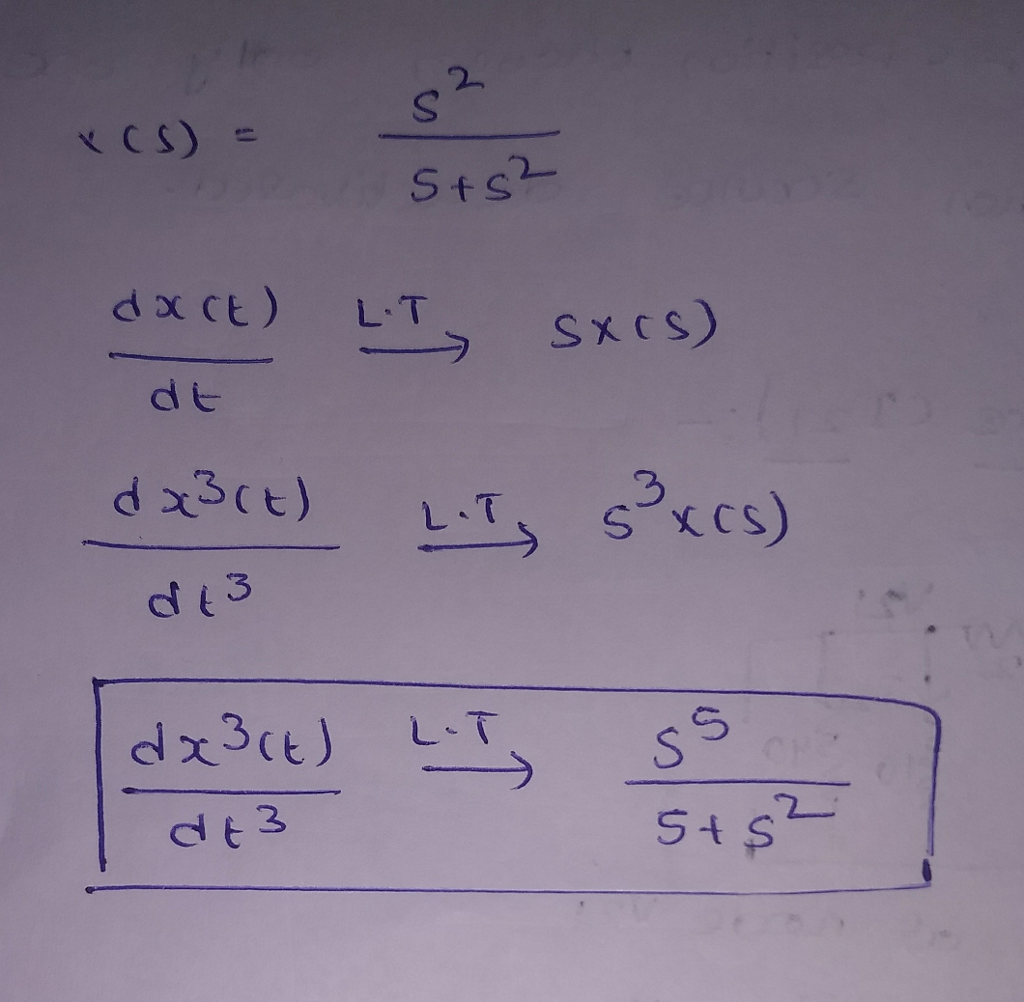#### Earn Coin

Coins can be redeemed for fabulous gifts.

Similar Homework Help Questions
• ### d) Find the Laplace transform of the following function: f (t = 0 to +09) eat dt e) Find the equivalent solution of (d) using MATLAB method(s) (find 2 methods). d) Find the Laplace transform...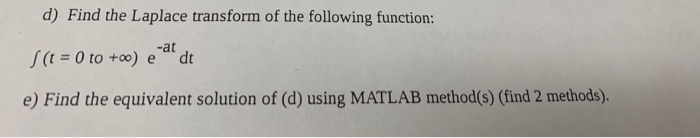d) Find the Laplace transform of the following function: f (t = 0 to +09) eat dt e) Find the equivalent solution of (d) using MATLAB method(s) (find 2 methods). d) Find the Laplace transform of the following function: f (t = 0 to +09) eat dt e) Find the equivalent solution of (d) using MATLAB method(s) (find 2 methods).

• ### Using laplace transform, for system described by: (d^2y(t))/dt^2 + (3dy(t))/dt + 2t(t) = dx(t)/dt - x...

Using laplace transform, for system described by: (d^2y(t))/dt^2 + (3dy(t))/dt + 2t(t) = dx(t)/dt - x (t) determine system transfer function and determine unit step response

• ### (5 points) Using the integral definition of the Laplace transform F(s) = Je-stf(t)dt, find the Laplaca...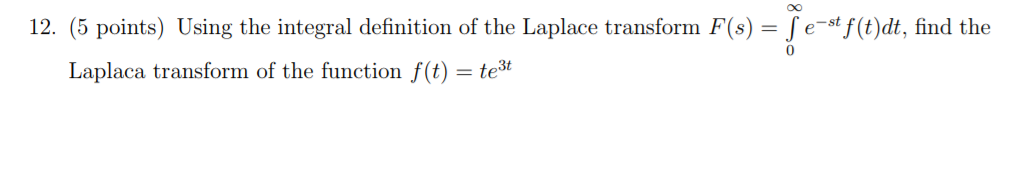(5 points) Using the integral definition of the Laplace transform F(s) = Je-stf(t)dt, find the Laplaca transform of the function f(t)-te 12

• ### Find the Laplace transform of f(t)=∫ 0 t τsin⁡(2τ) dτ F(s)= Find the Laplace transform of...Find the Laplace transform of f(t)=∫ 0 t τsin⁡(2τ) dτ F(s)= Find the Laplace transform of f(t) = Tsin(27) dt F() =

• ### slove the system eqution: d^3y(t)/dt^3 - 2 d^2y(t)/dt^2 - 5 dy(t)/dt +6 y(t) = 2 d^2u(t)/dt^2 +du(t)/dt +u(t) A) compute...

slove the system eqution: d^3y(t)/dt^3 - 2 d^2y(t)/dt^2 - 5 dy(t)/dt +6 y(t) = 2 d^2u(t)/dt^2 +du(t)/dt +u(t) A) compute the transfer function Y(s)/U(s)? B)Find inverse Laplace for y(t) and x(t)? C) find the final value of the system? D)find the initial value of the system? Please solve clearly with steps.

• ### Problem 1: Find the Laplace transform X(s) of x(0)-6cos(Sr-3)u(t-3). 10 Problem 2: (a) Find the inverse...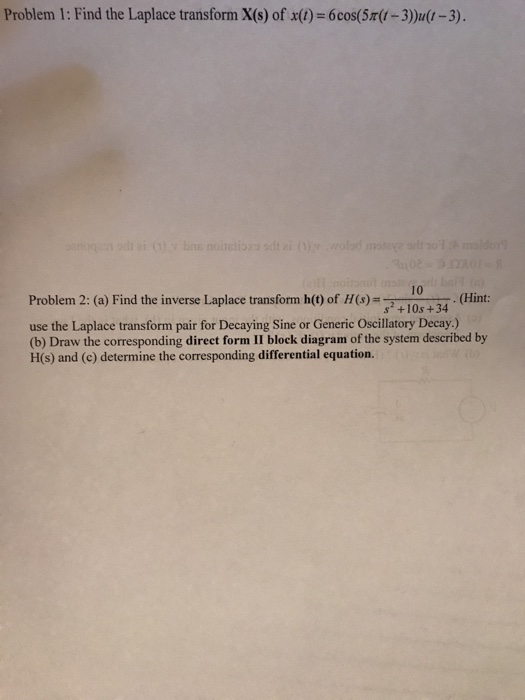Problem 1: Find the Laplace transform X(s) of x(0)-6cos(Sr-3)u(t-3). 10 Problem 2: (a) Find the inverse Laplace transform h() of H(s)-10s+34 (Hint: use the Laplace transform pair for Decaying Sine or Generic Oscillatory Decay.) (b) Draw the corresponding direct form II block diagram of the system described by H(s) and (c) determine the corresponding differential equation. Problem 3: Using the unilateral Laplace transform, solve the following differential equation with the given initial condition: y)+5y(0) 2u), y(0)1 Problem 4: For the...

• ### 3). Use the Laplace transform to solve for y(t) for t20. y(0 +) = 5, dt...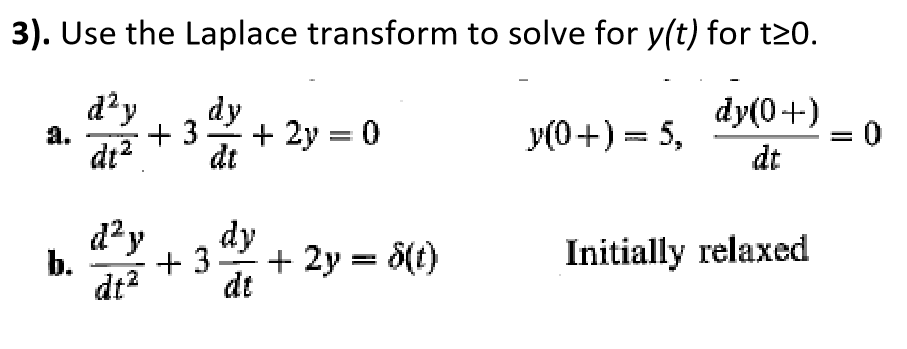3). Use the Laplace transform to solve for y(t) for t20. y(0 +) = 5, dt dt dt Initially relaxed dtdt

• ### A signal x(t) has the following Laplace transform X(s)= 2s+4 \$2+45+5 Get x(t) (inverse Laplace Transform)...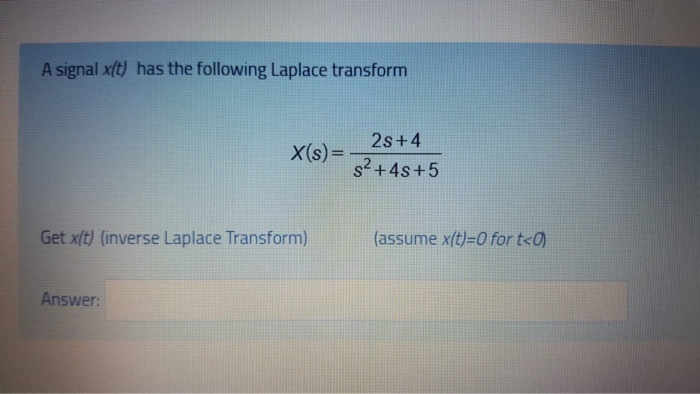A signal x(t) has the following Laplace transform X(s)= 2s+4 \$2+45+5 Get x(t) (inverse Laplace Transform) (assume x(t)=0 for t<0) Answer:

• ### Laplace Transform 3. If the ROC for a Laplace Transform pair x(t) <-> X(s) contains the...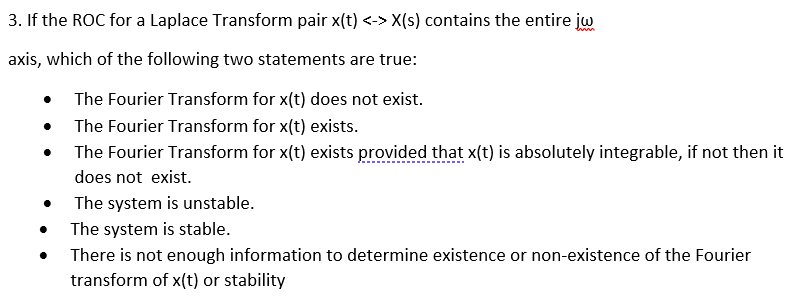Laplace Transform 3. If the ROC for a Laplace Transform pair x(t) <-> X(s) contains the entire w . axis, which of the following two statements are true: The Fourier Transform for x(t) does not exist. The Fourier Transform for x(t) exists. The Fourier Transform for x(t) exists provided that x(t) is absolutely integrable, if not then it does not exist. The system is unstable. The system is stable. There is not enough information to determine existence or non-existence of...

• ### do problem 2 and 4 Problem #2 Find the Laplace Transform 5t 2 3 Place Transform...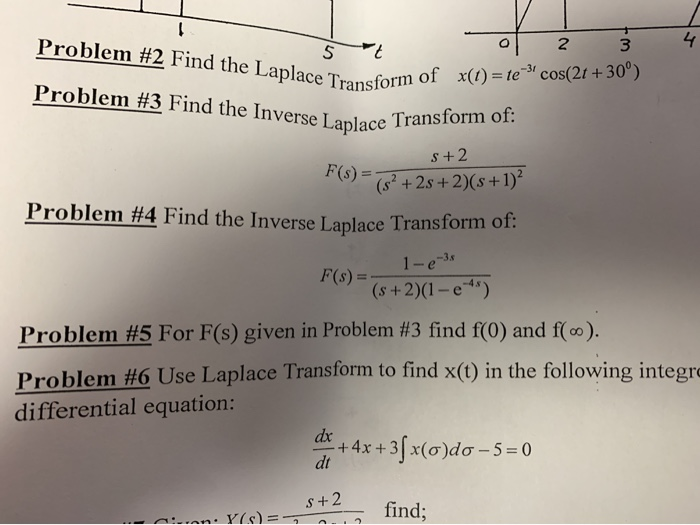do problem 2 and 4 Problem #2 Find the Laplace Transform 5t 2 3 Place Transform of X(t) = te-* cos(2t +30°) Problem #3 Find the Inverse Laplace Tran Tse Laplace Transform of: s+2 F(S) = (y2 +28+2)(s +1) Problem #4 Find the Inverse Laplace Transform 1-03 (s +2)(1 - e-*) F(s) = Problem #5 For F(s) given in Problem #3 find f(0) and f(co). Problem #6 Use Laplace Transform to find x(t) in the following integra differential equation: dx...